Publication date: 03/23/2021

## Power for One Sample Mean

Use the Power Explorer for One Sample Mean to determine a sample size for a hypothesis test about one mean. Select DOE > Sample Size Explorers > Power > Power for One Sample Mean. Explore the trade-offs between variability assumptions, sample size, power, significance, and the hypothesized difference to detect. Sample size and power are associated with the following hypothesis test: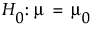versus the two-sided alternative: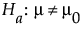or versus a one-sided alternative: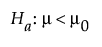or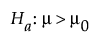where μ is the true mean and μ0 is the null mean or reference value. The difference to detect is an amount, Δ, away from μ0 that one considers important to detect. For the same significance level and power, a larger sample size is needed to detect a small difference than to detect a large difference. It is assumed that the population of interest is normally distributed with mean μ and standard deviation σ.

### Power Explorer for One Sample Mean Options

Set study assumptions and explore sample sizes using the radio buttons, text boxes, and sliders. The curve updates as you make changes to the settings. Alternatively, change settings by dragging the cross hairs on the curve or adjusting the values in the axis text boxes.

Test Type

Specifies a one or two-sided hypothesis test.

Fixed Parameters

Alpha

Specifies the probability of a type I error, which is the probability of rejecting the null hypothesis when it is true. It is commonly referred to as the significance level of the test. The default alpha level is 0.05.

Std Dev (σ)

Specifies the assumed standard deviation.

Tip: Use a standard deviation of 1 to estimate the sample size needed to detect differences measured in standard deviation units.

Population standard deviation known

Specifies use of calculations based on a known population standard deviation.

Test Parameters

Parameters that are inter-related and update as you make changes.

Difference to detect (Δ)

Specifies smallest difference between the true mean and the hypothesized or reference mean that you want to be able to declare statistically significant.

Sample Size

Specifies the total number of observations (runs, experimental units, or samples) needed for your experiment.

Power

Specifies the probability of rejecting the null hypothesis when it is false. With all other parameters fixed, power increases as sample size increases.

Save Settings

Saves the current settings to the Saved Settings table. This enables you to save a set of alternative study plans. See Saved Settings in the Sample Size Explorers.

Make Data Collection Table

Creates a new data table that you can use for data collection.

### Statistical Details for One Sample Power

The one sample mean calculations are based on the traditional t test when σ is unknown. For the case when σ is known, the calculations use the z test. For the case when σ is unknown, the power is calculated according to the alternative hypothesis.

For a one-sided, higher alternative: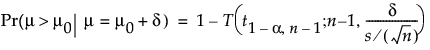For a one-sided, lower alternative: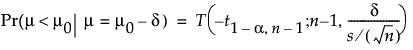For a two-sided alternative: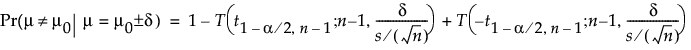where:

α is the significance level

n is the sample size

s is the standard deviation

δ is the difference to detect

t1-α,ν is the (1 - α)th quantile of the central t-distribution with ν degrees of freedom

T(t; ν, λ) is the cumulative distribution function of the non-central t distribution with ν degrees of freedom and non-centrality parameter λ.

When σ is known the z distribution and σ are used in the above equations for the power calculations. Because closed-form solutions for δ and n do not exist, numerical routines are used to solve for them.

Want more information? Have questions? Get answers in the JMP User Community (community.jmp.com).